Xlsxwriter 是一个用于编写 Excel 文件格式文件的 Python 模块，xlsxwriter 可以用来写文本，数字，公式和超链接到多个工作表，它支持的功能也有很多，譬如格式化、单元格合并、图标功能等，具体功能如下: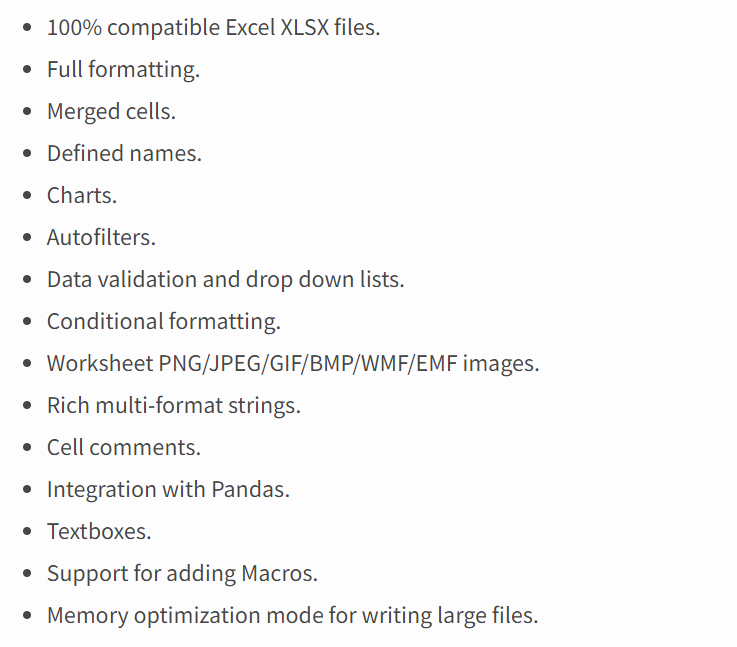xlsxwriter 同样也使用 pip 安装，安装语句如下：`pip install xlsxwriter`

### 简单使用

 `12345678910` `````` import xlsxwriter workbook = xlsxwriter.Workbook('demo.xlsx') # 建立文件 worksheet = workbook.add_worksheet() # 建立sheet， 可以使用work.add_worksheet('employee')来指定sheet名，如果命名中文名会报UnicodeDecodeErro的错误 worksheet.write('A1', 'Hello world') # 向A1写入文字 workbook.close() ``````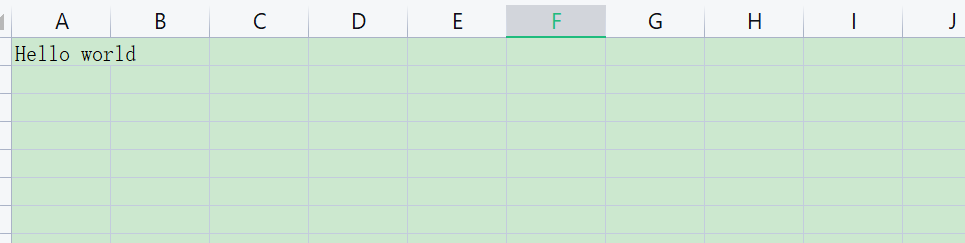### 实例

 `12345678910111213141516171819202122232425262728293031` ``````import xlsxwriter def simple_example(): # 创建一个新的Excel文件并添加一个工作表 workbook = xlsxwriter.Workbook('demo.xlsx') worksheet = workbook.add_worksheet() # 确定第一栏，使文字更清楚 worksheet.set_column('A:A', 20) # 添加粗体格式以突出显示单元格 bold = workbook.add_format({'bold': True}) # 简单的写一些文字 worksheet.write('A1', 'Hello') # 另起一行写入文字并加粗 worksheet.write('A2', 'World', bold) # 用行/列表示法写一些数字 worksheet.write(2, 0, 123) worksheet.write(3, 0, 13.432) # 插入一张图片. worksheet.insert_image('B5', 'logo.jpeg') workbook.close() if __name__ == '__main__': simple_example() ``````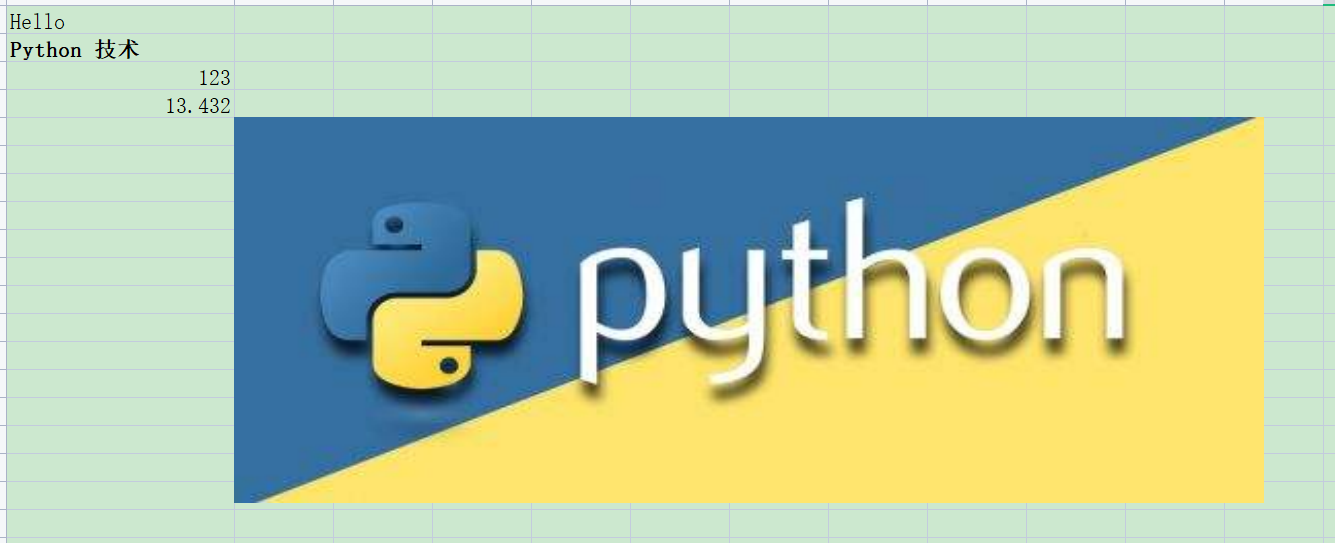### 表格数据求和

 `123456789101112131415161718192021222324` ``````def sum_data(): workbook = xlsxwriter.Workbook('demo.xlsx') # 建立文件 worksheet = workbook.add_worksheet() add_data = ( ['A1', 1087], ['A2', 1056], ['A3', 300], ['A4', 590], ) # 按标号写入是从0开始的，按绝对位置'A1'写入是从1开始的 row = 0 col = 0 # 遍历数据并逐行写出它 for item, cost in (add_data): worksheet.write(row, col, item) worksheet.write(row, col + 1, cost) row += 1 # 用公式写出总数 worksheet.write(row, 0, 'Total') worksheet.write(row, 1, '=SUM(B1:B4)') # 调用excel的公式表达式 workbook.close() ``````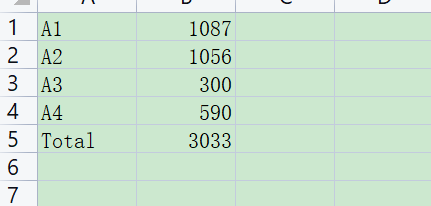### 自定义格式

 `1234567891011121314151617181920212223242526272829303132333435363738` ``````def self_define_format(): # 建文件及sheet. workbook = xlsxwriter.Workbook('demo2.xlsx') worksheet = workbook.add_worksheet() # Add a bold format to use to highlight cells. 设置粗体，默认是False bold = workbook.add_format({'bold': True}) # 定义数字格式 money = workbook.add_format({'num_format': '\$#,##0'}) # Write some data headers. 带自定义粗体blod格式写表头 worksheet.write('A1', 'Item', bold) worksheet.write('B1', 'Cost', bold) # Some data we want to write to the worksheet. add_data = ( ['A1', 1087], ['A2', 1056], ['A3', 300], ['A4', 590], ) # Start from the first cell below the headers. row = 1 col = 0 # Iterate over the data and write it out row by row. for item, cost in (add_data): worksheet.write(row, col, item) # 带默认格式写入 worksheet.write(row, col + 1, cost, money) # 带自定义money格式写入 row += 1 # Write a total using a formula. worksheet.write(row, 0, 'Total', bold) worksheet.write(row, 1, '=SUM(B2:B5)', money) workbook.close() ``````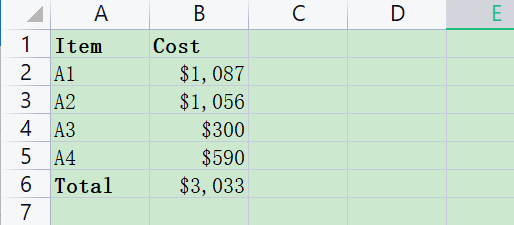### 写入日期格式

 `12345678910111213141516171819202122232425262728293031323334353637383940414243444546474849` ``````def write_date(): from datetime import datetime workbook = xlsxwriter.Workbook('demo3.xlsx') worksheet = workbook.add_worksheet() # 添加粗体格式以突出显示单元格. bold = workbook.add_format({'bold': 1}) # 为带钱的单元格添加数字格式. money_format = workbook.add_format({'num_format': '\$#,##0'}) # 添加Excel日期格式. date_format = workbook.add_format({'num_format': 'mmmm d yyyy'}) # 调整列的宽度 worksheet.set_column(1, 1, 15) # 写入数据表头 worksheet.write('A1', 'Item', bold) worksheet.write('B1', 'Date', bold) worksheet.write('C1', 'Cost', bold) # 将数据写入工作表 add_data = ( ['A1', '2013-01-13', 1875], ['A2', '2013-01-14', 345], ['A3', '2013-01-16', 564], ['A4', '2013-01-20', 10987], ) # 从标题下面的第一个单元格开始. row = 1 col = 0 for item, date_str, cost in (add_data): # 将日期字符串转换为datetime对象 date = datetime.strptime(date_str, "%Y-%m-%d") worksheet.write_string(row, col, item) worksheet.write_datetime(row, col + 1, date, date_format) worksheet.write_number(row, col + 2, cost, money_format) row += 1 # 用公式写出总数 worksheet.write(row, 0, 'Total', bold) worksheet.write(row, 2, '=SUM(C2:C5)', money_format) workbook.close() ``````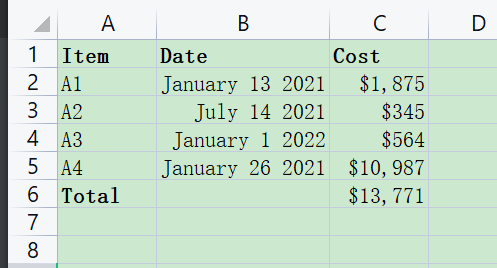### 参考

https://pypi.org/project/XlsxWriter/